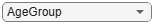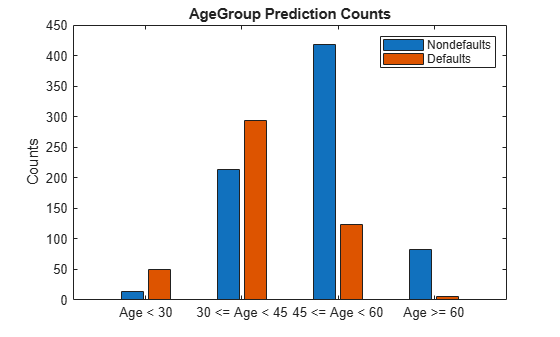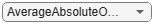# Explore Fairness Metrics for Credit Scoring Model

This example shows how to calculate and display fairness metrics for two sensitive attributes. You can use these metrics to test data and the model for fairness and then determine the thresholds to apply for your situation. You can also use the metrics to understand the biases in your model, the levels of disparity between groups, and how to assess the fairness of the model. This example uses the `fairnessMetrics` class in the Statistics and Machine Learning Toolbox$™$ to compute, display, and plot the various fairness metrics.

### Fairness Metrics Calculations

Fairness metrics are a set of measures that enable you to detect the presence of bias in your data or model. Bias refers to the preference of one group over another group, implicitly or explicitly. When you detect bias in your data or model, you can decide to take action to mitigate the bias. Bias detection is a set of measures that enable you to see the presence of unfairness toward one group or another. Bias mitigation is a set of tools to reduce the amount of bias that occurs in the data or model for the current analysis.

A set of metrics exists for the data and a set of metrics also exists for the model. Group metrics measure information within the group, whereas bias metrics measure differences across groups. The example calculates two bias metrics (Statistical Parity Difference (SPD) and Disparate Impact (DI)) and a group metric (group count) at the data level. In this example, you calculate four bias metrics and 17 group metrics at the model level.

Bias metrics:

• Statistical Parity Difference (SPD) measures the difference that the majority and protected classes receive a favorable outcome. This measure must be equal to 0 to be fair.

`$\mathrm{SPD}\text{\hspace{0.17em}}=\text{\hspace{0.17em}}\mathit{P}\left(\stackrel{ˆ}{\mathit{Y}}=1\text{\hspace{0.17em}}|\text{\hspace{0.17em}}\mathit{A}=\mathrm{minority}\right)-\mathit{P}\left(\stackrel{ˆ}{\mathit{Y}}=1\text{\hspace{0.17em}}|\text{\hspace{0.17em}}\mathit{A}=\mathrm{majority}\right),\mathrm{where}\text{\hspace{0.17em}}\stackrel{ˆ}{\mathit{Y}}\text{\hspace{0.17em}}\mathrm{are}\text{\hspace{0.17em}}\mathrm{the}\text{\hspace{0.17em}}\mathrm{model}\text{\hspace{0.17em}}\mathrm{predictions}\text{\hspace{0.17em}}\mathrm{and}\text{\hspace{0.17em}}\mathit{A}\text{\hspace{0.17em}}\mathrm{is}\text{\hspace{0.17em}}\mathrm{the}\text{\hspace{0.17em}}\mathrm{group}\text{\hspace{0.17em}}\mathrm{of}\text{\hspace{0.17em}}\mathrm{the}\text{\hspace{0.17em}}\mathrm{sensitive}\text{\hspace{0.17em}}\mathrm{attribute}.$`

• Disparate Impact (DI) compares the proportion of individuals that receive a favorable outcome for two groups, a majority group and a minority group. This measure must be equal to 1 to be fair.

`$\mathrm{DI}=\mathit{P}\left(\stackrel{ˆ}{\mathit{Y}}=1\text{\hspace{0.17em}}|\text{\hspace{0.17em}}\mathit{A}=\mathrm{minority}\right)\text{\hspace{0.17em}}/\text{\hspace{0.17em}}\mathit{P}\left(\stackrel{ˆ}{\mathit{Y}}=1\text{\hspace{0.17em}}|\text{\hspace{0.17em}}\mathit{A}=\mathrm{majority}\right),\mathrm{where}\text{\hspace{0.17em}}\stackrel{ˆ}{\mathit{Y}}\text{\hspace{0.17em}}\mathrm{are}\text{\hspace{0.17em}}\mathrm{the}\text{\hspace{0.17em}}\mathrm{model}\text{\hspace{0.17em}}\mathrm{predictions}\text{\hspace{0.17em}}\mathrm{and}\text{\hspace{0.17em}}\mathit{A}\text{\hspace{0.17em}}\mathrm{is}\text{\hspace{0.17em}}\mathrm{the}\text{\hspace{0.17em}}\mathrm{group}\text{\hspace{0.17em}}\mathrm{of}\text{\hspace{0.17em}}\mathrm{the}\text{\hspace{0.17em}}\mathrm{sensitive}\text{\hspace{0.17em}}\mathrm{attribute}.$`

• Equal Opportunity Difference (EOD) measures the deviation from the equality of opportunity, which means that the same proportion of each population receives the favorable outcome. This measure must be equal to 0 to be fair.

`$\mathrm{EOD}=\mathit{P}\left(\stackrel{ˆ}{\mathit{Y}}=1\text{\hspace{0.17em}}|\text{\hspace{0.17em}}\mathit{A}=\text{\hspace{0.17em}}\mathrm{minority},\mathit{Y}=1\right)-\mathit{P}\left(\stackrel{ˆ}{\mathit{Y}}=1\text{\hspace{0.17em}}|\text{\hspace{0.17em}}\mathit{A}=\text{\hspace{0.17em}}\mathrm{majority},\mathit{Y}=1\right),\mathrm{where}\text{\hspace{0.17em}}\stackrel{ˆ}{\mathit{Y}}\text{\hspace{0.17em}}\mathrm{are}\text{\hspace{0.17em}}\mathrm{the}\text{\hspace{0.17em}}\mathrm{model}\text{\hspace{0.17em}}\mathrm{predictions},\text{\hspace{0.17em}}\mathit{A}\text{\hspace{0.17em}}\mathrm{is}\text{\hspace{0.17em}}\mathrm{the}\text{\hspace{0.17em}}\mathrm{group}\text{\hspace{0.17em}}\mathrm{of}\text{\hspace{0.17em}}\mathrm{the}\text{\hspace{0.17em}}\mathrm{sensitive}\text{\hspace{0.17em}}\mathrm{attribute},\text{\hspace{0.17em}}\mathrm{and}\text{\hspace{0.17em}}\mathit{Y}\text{\hspace{0.17em}}\mathrm{are}\text{\hspace{0.17em}}\mathrm{the}\text{\hspace{0.17em}}\mathrm{true}\text{\hspace{0.17em}}\mathrm{labels}.$`

• Average Absolute Odds Difference (AAOD) measures bias by using the false positive rate and true positive rate. This measure must be equal to 0 to be fair.

`$\mathrm{AAOD}=\frac{1}{2}\left[|\mathit{FP}{\mathit{R}}_{\mathit{A}=\mathit{minority}}-\mathit{FP}{\mathit{R}}_{\mathit{A}=\mathit{majority}}|+|\mathit{TP}{\mathit{R}}_{\mathit{A}=\mathit{minority}}-\mathit{TP}{\mathit{R}}_{\mathit{A}=\mathit{majority}}|\right],\mathrm{where}\text{\hspace{0.17em}}\mathit{A}\text{\hspace{0.17em}}\mathrm{is}\text{\hspace{0.17em}}\mathrm{the}\text{\hspace{0.17em}}\mathrm{group}\text{\hspace{0.17em}}\mathrm{of}\text{\hspace{0.17em}}\mathrm{the}\text{\hspace{0.17em}}\mathrm{sensitive}\text{\hspace{0.17em}}\mathrm{attribute}.$`

Group metrics:

• True Positives (TP) is the total number of outcomes where the model correctly predicts the positive class.

• True Negatives (TN) is the total number of outcomes where the model correctly predicts the negative class.

• False Positives (FP) is the total number of outcomes where the model incorrectly predicts the positive class.

• False Negatives (FN) is the total number of outcomes where the model incorrectly predicts the negative class.

• True Positive Rate (TPR) is the sensitivity.

`$\mathrm{TPR}=\text{\hspace{0.17em}}\frac{\mathrm{TP}}{\left(\mathrm{TP}+\mathrm{FN}\right)}$`

• True Negative Rate (TNR) is the specificity or selectivity.

`$\mathrm{TNR}=\text{\hspace{0.17em}}\frac{\mathrm{TN}}{\left(\mathrm{TN}+\mathrm{FP}\right)}$`

• False Positive Rate (FPR) is the Type-I error.

`$\mathrm{FPR}=\text{\hspace{0.17em}}\frac{\mathrm{FP}}{\left(\mathrm{FP}+\mathrm{TN}\right)}$`

• False Negative Rate (FNR) is the Type-II error.

`$\mathrm{FNR}=\text{\hspace{0.17em}}\frac{\mathrm{FN}}{\left(\mathrm{FN}+\mathrm{TP}\right)}$`

• False Discovery Rate (FDR) is the ratio of the number of false positive results to the number of total positive test results.

`$\mathrm{FDR}=\text{\hspace{0.17em}}\frac{\mathrm{FP}}{\left(\mathrm{FP}+\mathrm{TP}\right)}$`

• False Omission Rate (FOR) is the ratio of the number of individuals with a negative predicted value for which the true label is positive.

`$\mathrm{FOR}=\text{\hspace{0.17em}}\frac{\mathrm{FN}}{\left(\mathrm{FN}+\mathrm{TN}\right)}$`

• Positive Predictive Value (PPV) is the ratio of the number of true positives to the number of true positives and false positives.

`$\mathrm{PPV}=\text{\hspace{0.17em}}\frac{\mathrm{TP}}{\left(\mathrm{TP}+\mathrm{FP}\right)}$`

• Negative Predictive Value (NPV) is the ratio of the number of true negatives to the number of true positives and false positives.

`$\mathrm{NPV}=\text{\hspace{0.17em}}\frac{\mathrm{TN}}{\left(\mathrm{TN}+\mathrm{FN}\right)}$`

• Rate of Positive Predictions (RPP) or Acceptance Rate is the ratio of the number of false and true positives to the total observations.

`$\mathrm{RPP}=\text{\hspace{0.17em}}\frac{\left(\mathrm{FP}+\mathrm{TP}\right)}{\left(\mathrm{TN}+\mathrm{TP}+\mathrm{FN}+\mathrm{FP}\right)}$`

• Rate of Negative Predictions (RNP) is the ratio of the number of false and true negatives to the total observations.

`$\mathrm{RNP}=\text{\hspace{0.17em}}\frac{\left(\mathrm{FN}+\mathrm{TN}\right)}{\left(\mathrm{TN}+\mathrm{TP}+\mathrm{FN}+\mathrm{FP}\right)}$`

• Accuracy (ACC) is the ratio of the number of true negatives and true positives to the total observations.

`$\mathrm{ACC}=\text{\hspace{0.17em}}\frac{\left(\mathrm{TN}+\mathrm{TP}\right)}{\left(\mathrm{TN}+\mathrm{TP}+\mathrm{FN}+\mathrm{FP}\right)}$`

• Group Count is the number of individuals in the group.

• Group Size Ratio is the ratio of the number of individuals in that group to the total number of individuals.

The example focuses on bias detection in credit card data and explores bias metrics and group metrics based on the sensitive attributes of customer age (`CustAge`) and residential status (`ResStatus`). The data contains the residential status as a categorical variable and the customer age as a numeric variable. To create predictions and analyze the data for fairness, you group the customer age variable into bins.

### Visualize Sensitive Attributes in Credit Card Data

Load the credit card data set. Group the customer age into bins. Use the `discretize` function for a numeric variable to create groups that identify age groups of interest for comparison on fairness. Retrieve the counts for both sensitive attributes of customer age and residential status.

```load CreditCardData.mat AgeGroup = discretize(data.CustAge,[min(data.CustAge) 30 45 60 max(data.CustAge)], ... 'categorical',{'Age < 30','30 <= Age < 45','45 <= Age < 60','Age >= 60'}); data = addvars(data,AgeGroup,'After','CustAge'); gs_data_ResStatus = groupsummary(data,{'ResStatus','status'}); gs_data_AgeGroup = groupsummary(data,{'AgeGroup','status'}); ```

Plot the count of customers who have defaulted on their credit card payments and who have not defaulted by age.

```Attribute ="AgeGroup"; figure bar(unique(data.(Attribute)), ... [eval("gs_data_"+Attribute+".GroupCount(1:2:end)"), ... eval("gs_data_"+Attribute+".GroupCount(2:2:end)")]'); title(Attribute +" True Counts"); ylabel('Counts') legend({'Nondefaults','Defaults'})```### Calculate Fairness Metrics for Data

Calculate fairness metrics for the residential status and customer age data. The `fairnessMetrics` class returns a `fairnessMetrics` object, whichis then passed into the `report` method to obtain a table with bias metrics and group metrics. Bias metrics take into account two classes (the majority and minority) at a time, while group metrics are within the individual group. In the data set, if you use residential status as the sensitive attribute, then the `Home Owner` group is the majority class because this class contains the largest number of individuals. Based on the SPD and DI metrics, the data set does not show a significant presence of bias for residential status. For the customer age data, the age group between 45 and 60 is the majority class because this class contains the largest number of individuals. Compared to the residential status, based on the SPD and DI metrics, the age group that is greater than 60 shows a slightly larger presence of bias.

`dataMetricsObj = fairnessMetrics(data, 'status', 'SensitiveAttributeNames',{'ResStatus','AgeGroup'})`
```dataMetricsObj = fairnessMetrics with properties: SensitiveAttributeNames: {'ResStatus' 'AgeGroup'} ReferenceGroup: {'Home Owner' '45 <= Age < 60'} ResponseName: 'status' PositiveClass: 1 BiasMetrics: [7x4 table] GroupMetrics: [7x4 table] ```
`dataMetricsTable = report(dataMetricsObj,'GroupMetrics','GroupCount')`
```dataMetricsTable=7×5 table SensitiveAttributeNames Groups StatisticalParityDifference DisparateImpact GroupCount _______________________ ______________ ___________________________ _______________ __________ ResStatus Home Owner 0 1 542 ResStatus Tenant 0.025752 1.0789 474 ResStatus Other -0.038525 0.88203 184 AgeGroup Age < 30 0.0811 1.2759 64 AgeGroup 30 <= Age < 45 0.10333 1.3516 506 AgeGroup 45 <= Age < 60 0 1 541 AgeGroup Age >= 60 -0.14783 0.497 89 ```

### Create Credit Scorecard Model and Generate Predictions

Create a credit scorecard model using the `creditscorecard` function. Perform automatic binning of the predictors using the `autobinning` function. Fit a logistic regression model to the Weight of Evidence (WOE) data using the `fitmodel` function. Store the predictor names and corresponding coefficients in the credit scorecard model.

```PredictorVars = setdiff(data.Properties.VariableNames, ... {'AgeGroup','CustID','status'}); sc = creditscorecard(data,'IDVar','CustID', ... 'PredictorVars',PredictorVars); sc = autobinning(sc); sc = fitmodel(sc);```
```1. Adding CustIncome, Deviance = 1490.8527, Chi2Stat = 32.588614, PValue = 1.1387992e-08 2. Adding TmWBank, Deviance = 1467.1415, Chi2Stat = 23.711203, PValue = 1.1192909e-06 3. Adding AMBalance, Deviance = 1455.5715, Chi2Stat = 11.569967, PValue = 0.00067025601 4. Adding EmpStatus, Deviance = 1447.3451, Chi2Stat = 8.2264038, PValue = 0.0041285257 5. Adding CustAge, Deviance = 1441.994, Chi2Stat = 5.3511754, PValue = 0.020708306 6. Adding ResStatus, Deviance = 1437.8756, Chi2Stat = 4.118404, PValue = 0.042419078 7. Adding OtherCC, Deviance = 1433.707, Chi2Stat = 4.1686018, PValue = 0.041179769 Generalized linear regression model: status ~ [Linear formula with 8 terms in 7 predictors] Distribution = Binomial Estimated Coefficients: Estimate SE tStat pValue ________ ________ ______ __________ (Intercept) 0.70239 0.064001 10.975 5.0538e-28 CustAge 0.60833 0.24932 2.44 0.014687 ResStatus 1.377 0.65272 2.1097 0.034888 EmpStatus 0.88565 0.293 3.0227 0.0025055 CustIncome 0.70164 0.21844 3.2121 0.0013179 TmWBank 1.1074 0.23271 4.7589 1.9464e-06 OtherCC 1.0883 0.52912 2.0569 0.039696 AMBalance 1.045 0.32214 3.2439 0.0011792 1200 observations, 1192 error degrees of freedom Dispersion: 1 Chi^2-statistic vs. constant model: 89.7, p-value = 1.4e-16 ```

Display unscaled points for predictors retained in the model using the `displaypoints` function.

`pointsinfo = displaypoints(sc)`
```pointsinfo=37×3 table Predictors Bin Points ______________ ________________ _________ {'CustAge' } {'[-Inf,33)' } -0.15894 {'CustAge' } {'[33,37)' } -0.14036 {'CustAge' } {'[37,40)' } -0.060323 {'CustAge' } {'[40,46)' } 0.046408 {'CustAge' } {'[46,48)' } 0.21445 {'CustAge' } {'[48,58)' } 0.23039 {'CustAge' } {'[58,Inf]' } 0.479 {'CustAge' } {'<missing>' } NaN {'ResStatus' } {'Tenant' } -0.031252 {'ResStatus' } {'Home Owner' } 0.12696 {'ResStatus' } {'Other' } 0.37641 {'ResStatus' } {'<missing>' } NaN {'EmpStatus' } {'Unknown' } -0.076317 {'EmpStatus' } {'Employed' } 0.31449 {'EmpStatus' } {'<missing>' } NaN {'CustIncome'} {'[-Inf,29000)'} -0.45716 ⋮ ```

For details about creating a more in depth credit scoring model, see the Bin Data to Create Credit Scorecards Using Binning Explorer.

Calculate the probability of default for the credit scorecard model using the `probdefault` function. Define the threshold for the probability of default as 0.35. Create an array of predictions where each value is greater than the threshold.

```pd = probdefault(sc); threshold = 0.35; predictions = double(pd>threshold);```

Add the resulting predictions to the `data` output table. To calculate bias metrics, you can set aside a set of validation data. Retrieve the counts for the residential status and customer age predictions. Plot the customer age predictions.

```data = addvars(data,predictions,'After','status'); gs_predictions_ResStatus = groupsummary(data,{'ResStatus','predictions'}, ... 'IncludeEmptyGroups',true); gs_predictions_AgeGroup = groupsummary(data,{'AgeGroup','predictions'}, ... 'IncludeEmptyGroups',true); Attribute ="AgeGroup"; figure bar(unique(data.(Attribute)), ... [eval("gs_predictions_"+Attribute+".GroupCount(1:2:end)"), ... eval("gs_predictions_"+Attribute+".GroupCount(2:2:end)")]'); title(Attribute +" Prediction Counts"); ylabel('Counts') legend({'Nondefaults','Defaults'})```### Calculate and Visualize Fairness Metrics for Credit Scorecard Model

Calculate model bias and group metrics for residential status and customer age. For the DI model metric, the commonly used range to assess fairness is between 0.8 and 1.25 . A value of less than 0.8 indicates the presence of bias. However, a value greater than 1.25 indicates that something is incorrect and additional investigation might be required. The model bias metrics in this example show a greater effect on fairness than the data bias metrics. After the model has been fitted, the negative SPD and EOD values mean that the `Other` group shows a slight presence of bias. In the group metrics, the FPR group metric of 39.7% is higher for tenants than home owners, which means that tenants are more likely to be falsely labeled as defaults. The FDR, FOR, PPV, and NPV group metrics show a very minimal presence of bias.

Looking at the model bias metrics SPD, DI, EOD, and AAOD for customer age, the 30 and under group has the greatest variance from the majority class and might require further investigation. Further, the age group over 60 shows the presence of bias based on the negative SPD and EOD values and the very low DI value. Also, based on the DI metrics, additional model bias mitigation might be required.

In the group metrics, the FPR group metric of 80% is much higher for the 30 and under group than the majority class, which means that those individuals whose age is 30 and under are more likely to be falsely labeled as defaults. The FDR group metric of 83.3% is much higher for the over 60 group than the majority class, which means that 83.3% of individuals whose age is over 60 and identified as defaults by the model are false positives. The `Accuracy` metric shows the highest accuracy for the over 60 group at 80.9%.

`modelMetricsObj = fairnessMetrics(data, 'status', 'SensitiveAttributeNames',{'ResStatus','AgeGroup'},'Predictions',predictions)`
```modelMetricsObj = fairnessMetrics with properties: SensitiveAttributeNames: {'ResStatus' 'AgeGroup'} ReferenceGroup: {'Home Owner' '45 <= Age < 60'} ResponseName: 'status' PositiveClass: 1 BiasMetrics: [7x6 table] GroupMetrics: [7x19 table] ```
`modelMetricsTable = report(modelMetricsObj,'GroupMetrics','all')`
```modelMetricsTable=7×23 table SensitiveAttributeNames Groups StatisticalParityDifference DisparateImpact EqualOpportunityDifference AverageAbsoluteOddsDifference GroupCount GroupSizeRatio TruePositives TrueNegatives FalsePositives FalseNegatives TruePositiveRate TrueNegativeRate FalsePositiveRate FalseNegativeRate FalseDiscoveryRate FalseOmissionRate PositivePredictiveValue NegativePredictiveValue RateOfPositivePredictions RateOfNegativePredictions Accuracy _______________________ ______________ ___________________________ _______________ __________________________ _____________________________ __________ ______________ _____________ _____________ ______________ ______________ ________________ ________________ _________________ _________________ __________________ _________________ _______________________ _______________________ _________________________ _________________________ ________ ResStatus Home Owner 0 1 0 0 542 0.45167 88 252 113 89 0.49718 0.69041 0.30959 0.50282 0.56219 0.261 0.43781 0.739 0.37085 0.62915 0.62731 ResStatus Tenant 0.10173 1.2743 0.1136 0.1007 474 0.395 102 185 122 65 0.61078 0.60261 0.39739 0.38922 0.54464 0.26 0.45536 0.74 0.47257 0.52743 0.60549 ResStatus Other -0.11541 0.68878 -0.082081 0.10042 184 0.15333 22 106 25 31 0.41509 0.80916 0.19084 0.58491 0.53191 0.22628 0.46809 0.77372 0.25543 0.74457 0.69565 AgeGroup Age < 30 0.55389 3.4362 0.41038 0.51487 64 0.053333 18 8 32 6 0.75 0.2 0.8 0.25 0.64 0.42857 0.36 0.57143 0.78125 0.21875 0.40625 AgeGroup 30 <= Age < 45 0.35169 2.5469 0.35192 0.3381 506 0.42167 139 151 154 62 0.69154 0.49508 0.50492 0.30846 0.5256 0.29108 0.4744 0.70892 0.57905 0.42095 0.57312 AgeGroup 45 <= Age < 60 0 1 0 0 541 0.45083 54 313 69 105 0.33962 0.81937 0.18063 0.66038 0.56098 0.2512 0.43902 0.7488 0.22736 0.77264 0.67837 AgeGroup Age >= 60 -0.15994 0.29652 -0.2627 0.18877 89 0.074167 1 71 5 12 0.076923 0.93421 0.065789 0.92308 0.83333 0.14458 0.16667 0.85542 0.067416 0.93258 0.80899 ```

Choose the bias metric and sensitive attribute and plot it. This code selects `AAOD` and `AgeGroup` by default.

```BiasMetric ="AverageAbsoluteOddsDifference"; SensitiveAttribute ="AgeGroup"; plot(modelMetricsObj, BiasMetric, "SensitiveAttributeNames", SensitiveAttribute);```For the same sensitive attribute, choose the group metric and plot it. This code selects the group count by default. The resulting plots show the metric values for the selected sensitive attribute.

```GroupMetric ="GroupCount"; plot(modelMetricsObj, GroupMetric, "SensitiveAttributeNames", SensitiveAttribute);```

Plot the SPD, DI, EOD, and AAOD bias metrics for the two sensitive attributes.

```MetricsShort = ["spd" "di" "eod" "aaod"]; tiledlayout(2,4)``````for sa = string(modelMetricsObj.SensitiveAttributeNames) for m = MetricsShort nexttile h = plot(modelMetricsObj,m,'SensitiveAttributeNames',sa); title(h.Parent,upper(m)); h.Parent.XLabel = []; if m~=MetricsShort(1) h.Parent.YTickLabel = ''; h.Parent.YLabel = []; end end end```Bias preserving metrics seek to keep the historic performance in the outputs of a target model with equivalent error rates for each group as shown in the training data. These metrics do not alter the status quo that exists in society. A fairness metric is classified as bias preserving when a perfect classifier exactly satisfies the metric. In contrast, bias transforming metrics require the explicit decision regarding which biases the system should exhibit. These metrics do not accept the status quo and acknowledge that protected groups start from different points that are not equal. The main difference between these two types of metrics is that most bias transforming metrics are satisfied by matching decision rates between groups, whereas bias preserving metrics require matching error rates instead. To assess the fairness of a decision-making system, use both bias preserving and transforming metrics to create the broadest possible view of the bias in the system.

Evaluating whether a metric is bias preserving is straightforward with a perfect classifier. In the absence of a perfect classifier, you can substitute the predictions with the classifier response and observe if the formula is trivially true. EOD and AAOD are bias preserving metrics because they have no variance; however, SPD and DI are bias transforming metrics as they show a variance from the majority classes.

```biasMetrics_ResStatus1Obj = fairnessMetrics(data, 'status', 'SensitiveAttributeNames' ,'ResStatus', 'Predictions', 'status'); report(biasMetrics_ResStatus1Obj)```
```ans=3×6 table SensitiveAttributeNames Groups StatisticalParityDifference DisparateImpact EqualOpportunityDifference AverageAbsoluteOddsDifference _______________________ __________ ___________________________ _______________ __________________________ _____________________________ ResStatus Home Owner 0 1 0 0 ResStatus Tenant 0.025752 1.0789 0 0 ResStatus Other -0.038525 0.88203 0 0 ```
```biasMetrics_AgeGroup1Obj = fairnessMetrics(data, 'status', 'SensitiveAttributeNames', 'AgeGroup', 'Predictions', 'status'); report(biasMetrics_AgeGroup1Obj)```
```ans=4×6 table SensitiveAttributeNames Groups StatisticalParityDifference DisparateImpact EqualOpportunityDifference AverageAbsoluteOddsDifference _______________________ ______________ ___________________________ _______________ __________________________ _____________________________ AgeGroup Age < 30 0.0811 1.2759 0 0 AgeGroup 30 <= Age < 45 0.10333 1.3516 0 0 AgeGroup 45 <= Age < 60 0 1 0 0 AgeGroup Age >= 60 -0.14783 0.497 0 0 ```

### References

1. Schmidt, Nicolas, Sue Shay, Steve Dickerson, Patrick Haggerty, Arjun R. Kannan, Kostas Kotsiopoulos, Raghu Kulkarni, Alexey Miroshnikov, Kate Prochaska, Melanie Wiwczaroski, Benjamin Cox, Patrick Hall, and Josephine Wang. Machine Learning: Considerations for Fairly and Transparently Expanding Access to Credit. Mountain View, CA: H2O.ai, Inc., July 2020.

2. Mehrabi, Ninareh, et al. “A Survey on Bias and Fairness in Machine Learning.” ArXiv:1908.09635 [Cs], Sept. 2019. arXiv.org, https://arxiv.org/abs/1908.09635.

3. Saleiro, Pedro, et al. “Aequitas: A Bias and Fairness Audit Toolkit.” ArXiv:1811.05577 [Cs], Apr. 2019. arXiv.org, https://arxiv.org/abs/1811.05577.

4. Wachter, Sandra, et al. Bias Preservation in Machine Learning: The Legality of Fairness Metrics Under EU Non-Discrimination Law. SSRN Scholarly Paper, ID 3792772, Social Science Research Network, 15 Jan. 2021. papers.ssrn.com, https://papers.ssrn.com/sol3/papers.cfm?abstract_id=3792772.